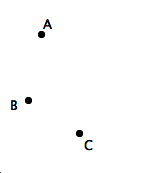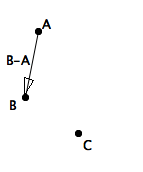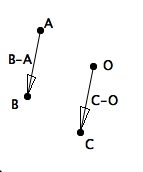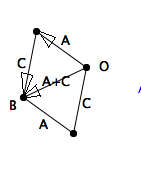Vector Comic 1
4jan14

\begin{document}
\maketitleSuppose we have 3 points $A,B,C$ and are told that $C=B-A$.This means that $B-A = \vec{AB}$.Because $C = C-O$, where $O$ is the origin, we can infer where the origin is located.Vector algebra says that $B=A+C$, which is clear once we complete the traditional vector addition paralellogram.

Note that $B= A + (B-A)$ also. That means we can get to $B$ by starting
at
$A$ and following along the displacement vector $B-A$, which we have
renamed $C$ in the original hypothesis.

Thus we have presented geometrical interpretations of some simple
algebra.
\end{document}